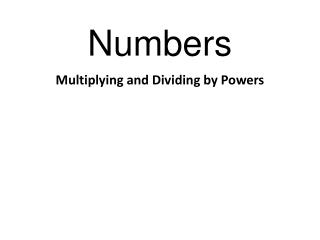DownloadDownload PresentationNumbers

# Numbers

Télécharger la présentation## Numbers

- - - - - - - - - - - - - - - - - - - - - - - - - - - E N D - - - - - - - - - - - - - - - - - - - - - - - - - - -
##### Presentation Transcript

1. Numbers Multiplying and Dividing by Powers

2. Numbers Any number (or letter) multiplied by itself will result in a square number. 2 x 2 = 4, 5 x 5 = 25 We call it a square number because …

3. Numbers …the area of a square is the length multiplied by the width, and these are always the same…obviously! 2cm x 2cm = 4cm2 2cm 2cm

4. Numbers Instead of writing 2 x 2 (or the answer 4), in maths we write a number that is going to be squared like this: 22 We call this the BASE number

5. Numbers Instead of writing 2 x 2 (or the answer 4), in maths we write a number that is going to be squared like this: 22 And we call this the POWER or INDICES

6. Numbers The same is true in algebra. When we need to multiply a letter by itself instead of writing a x a we write a2

7. Numbers If we have to multiply a number by itself 3 times, or 4 times , then the small number (the power or indices) increases to show us how many times we multiply the number by itself… 4 x 4 x 4 = 7 x 7 x 7 x 7 x 7 x 7 = 43 76

8. Numbers g x g x g = g3 What happens if we multiply g3 by g2? Do we get… IT ALL GETS SO CONFUSING!!!! 2g5 or 2g6 or g5 or g6

9. Numbers We need to understand how this works g3 = g x g x g g2 = g x g So g3 x g2 is the same as g x g x g xg x g Which is… g5

10. Numbers 212 From this we can now say that when we multiply power numbers we add the indices together: 24 x 28 = 33 x 35 = t2 x t8 = 38 t10

11. Numbers If we add indices when we multiply power numbers, what do we do when we divide power numbers? Subtract the indices! 28 ÷ 22 = 39÷ 34 = T6 ÷ t4 = 26 35 t2

12. Numbers Just two things to be careful of. y x y4 = y5 because we do not put a 1 next to a base number. 63 ÷ 63 = 1 Because 6 goes into 6 once, 6x6x6 (216) goes into 6x6x6 (216) once. Any number divided by itself is 1.